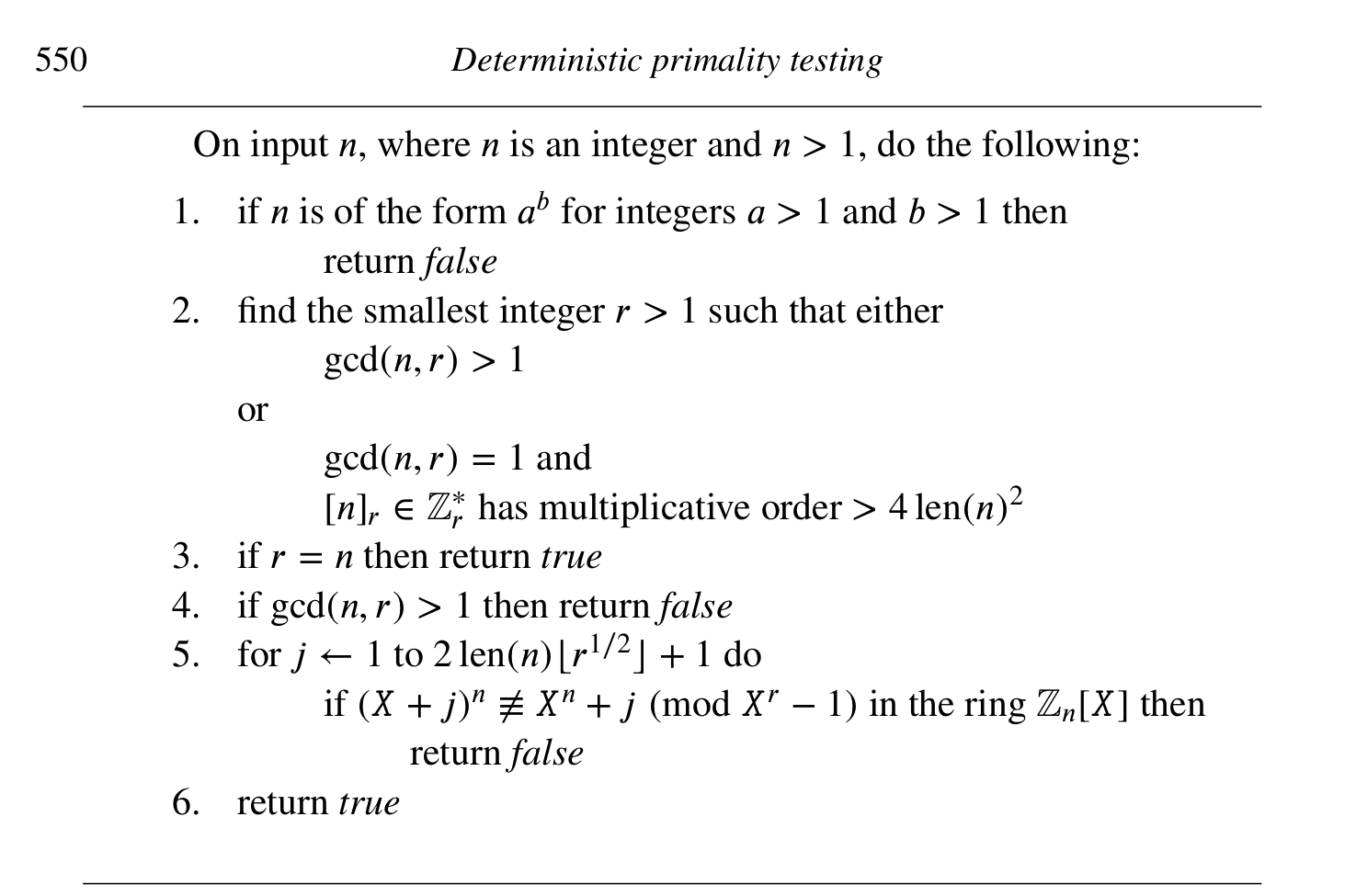# Implementing the AKS primality test

I am implementing the infamous AKS deterministic primality test using SageMath.

My code is as follows:

# AKS Primality Test
def fast_exponentation(a, n):
"""
Computes a^n fast
"""
ans = 1
while n:
if n & 1 : ans = ans * a
a = a * a
n >>= 1
return ans

# Determines whether n is a power a ^ b, b > 1
def is_perfect_power(n):
lgn = 1 + ( len( bin ( abs ( n ) ) ) - 2)
for b in range(2,lgn):
# we use binary search to check if n root b is an integer
lowa = 0
higha = n
while lowa < higha - 1:
mida = (lowa + higha) // 2
ab = fast_exponentation(mida,b)
if   ab > n: higha = mida
elif ab < n: lowa  = mida
else:        return True # mida ^ b
return False

def AKS_primality_test(n):
if n == 1: return None
if n == 2: return True

# if n is of the form a^b for a > 1 and b > 1 return False
if is_perfect_power(n): return False
print("Passed perfect power test")
# find the smallest integer r such that
#     a. gcd(n,r) > 1 or
#     b. [n]_r has multiplicative order > 4 log2(n)^2
for r in xrange(2,n):
# if r shares a non trivial factor with n, n is composite
if gcd(r,n) > 1: return False
# compute the multiplicative order of n in Z mod r
ord_r = mod(n,r).multiplicative_order()
if ord_r > 4*log(n,2)^2: break

print("min r found! r={}".format(r))

if r == n: return True

# We get the ring where we will check for primality
ZnX = IntegerModRing(n)[x]
ZnX_div_f = ZnX.quo(x^r-1)

for j in range(1, 2*log(n,2)*sqrt(r)+2):
if ZnX_div_f((x+j)^n) != ZnX_div_f(x^n+j):
print("The freshman dream test failed for j={}".format(j))
return False

print("Passed final test")

return True

print("271 is prime = " + str(AKS_primality_test(271)))


For the implementation I am following the scheme in page 550 of A Computational Introduction to Number Theory and Algebra, by Victor Shoup (available online in his webpage).However, the code above, which should test whether 271 is prime, gives us the following output:

Passed perfect power test
min r found! r=269
The freshman dream test failed for j=1
271 is prime = False


This is wrong - 271 is in fact prime. So I assume I am misunderstanding how to use quotient rings in SageMath.

Why does the equality test fail?

EDIT: as pointed out by @rburing, the line if ZnX_div_f((x+j)^n) != ZnX_div_f(x+j^n): should read if ZnX_div_f((x+j)^n) != ZnX_div_f(x^n+j): I have edited the code above to reflect this, but the behavior of the program is the same nonetheless.

edit retag close merge delete

Sort by » oldest newest most votedYou have a typo in your inner if-statement: x + j^n instead of x^n + j, so it always fails for degree reasons.

You probably also want to avoid using the symbolic x and instead give the generator of your polynomial ring a proper name such as x, and name the image in the quotient something like xbar:

# We get the ring where we will check for primality
ZnX.<x> = IntegerModRing(n)[]
ZnX_mod_f.<xbar> = ZnX.quo(x^r-1)

for j in range(1, 2*log(n,2)*sqrt(r)+2):
if (xbar+j)^n != xbar^n+j:
print("The freshman dream test failed for j={}".format(j))
return False

more

Good catch, thank you! Unfortunately, I get the same behavior after correcting the typo